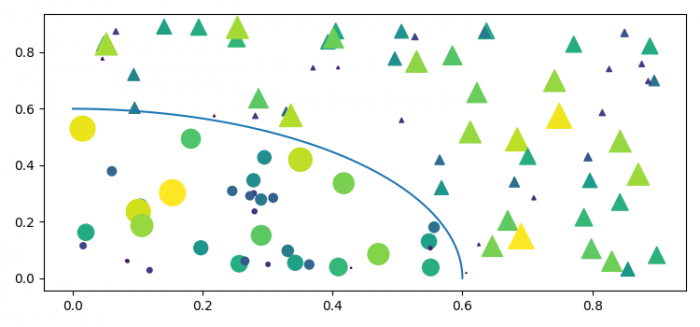To plot scattered masked points and add a line to demark the masked regions, we can take the following steps.

## Steps

• Set the figure size and adjust the padding between and around the subplots.
• Create N, r0, x, y, area, c, r, area1and area2 data points using numpy.
• Plot x and y data points using scatter() method.
• To demark the maked regions, plot the curve using plot() method.
• To display the figure, use show() method.

## Example

import matplotlib.pyplot as plt
import numpy as np

plt.rcParams["figure.figsize"] = [7.50, 3.50]
plt.rcParams["figure.autolayout"] = True

N = 100
r0 = 0.6
x = 0.9 * np.random.rand(N)
y = 0.9 * np.random.rand(N)
area = (20 * np.random.rand(N))**2
c = np.sqrt(area)

r = np.sqrt(x ** 2 + y ** 2)
area1 = np.ma.masked_where(r < r0, area)
area2 = np.ma.masked_where(r >= r0, area)
plt.scatter(x, y, s=area1, marker='^', c=c)
plt.scatter(x, y, s=area2, marker='o', c=c)
theta = np.arange(0, np.pi / 2, 0.01)
plt.plot(r0 * np.cos(theta), r0 * np.sin(theta))

plt.show()

## Output